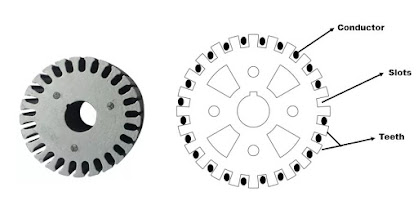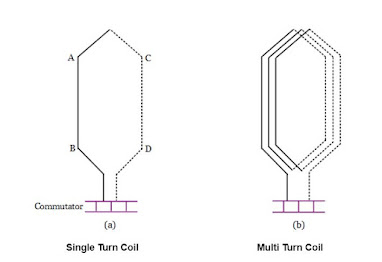# Armature winding | Terms Related to Armature winding | lap winding of dc generator | Wave winding of dc generator

## Armature Winding

Armature winding is an arrangement of conductors around the periphery of armature to develop desired emfs by relative motion in a hetero-polar magnetic field. In armature winding, conductors or group of conductors are distributed in different ways in slots all over the periphery of the armature. The conductors may be connected in series and parallel combinations depending upon the current and voltage rating of the machine.Armature Lamination

## Terms Related To Armature Windingfigure 1

The Various terms related to armature winding are discussed below

1. Conductor:  Each individual length of wire lying within the magnetic field is called conductor. it is denoted by “Z”.
in figure 1 (a) side "AB" and "CD" represents conductors.

2. Turn: when two conductor lying in  magnetic field are connected in series so that emf induced in them help each other. It is known as a turn.  Z = 2T.
Figure 1 (a) represents single turn coil, Z = 2 T = 2(1) = 2
Thus in single turn coil number conductors will be two ( i.e. "AB" and "CD")

Figure 1 (b) is multi turn coil. The coil has three turns, thus number of conductor will be
Z = 2T = 2(3) = 6

3. Coil: when one or more turns are connected in series and the two ends of it are connected to adjacent commutator segments (in lap winding) it is termed as a coil.To get maximum emf induced from a coil the emf induced in conductors of a coil should be should opposite to each other.
Number coils = number of slots (in case double layer winding)

There are two type of armature windings depending upon number of coil sides per slot - single layer winding and double layer winding.

single layer winding:-  It is the armature winding in which one coil side is placed on each armature slot, as shown in figure below
Double layer winding:- It is the armature winding in which two coil side are placed on each armature slot. One coil side of a coil lies at the top of a slot and the other coil side lies at the bottom of some other slot. as shown in figure below.Double Layer Winding

4. Pole Pitch:- It is defined as number of armature conductors or armature slots per pole. if a 4 pole Machine has 24 armature slots. Each slot contains two conductors.

Pole pitch in terms of Slots

Pole Pitch = \frac{24}{4}= 6

Pole pitch in terms of conductors

Pole Pitch = \frac{24\times 2}{4}= \frac{48}{4}=12

Sometimes the armature slots or number of conductors are not given. The diameter of bore are given.
Pole pitch in terms of diameter of bore

Pole Pitch =  \frac{\pi D}{P}

Where D = Diameter of bore

Diameter of bore = Diameter of armature + 2 ( Air gap )

5. Coil Span:- the Number of Armature Slots or armature conductors between two sides of coil. It is denoted by “Ys”.

If the coil span is 9 slots, it means one side of the coil is in slot 1 and the other side in slot 10.

If the coil span or coil pitch is equal to the pole pitch, the coil is said to be full pitch coil. It means coil span 180 electrical degrees i.e coil sides lie in opposite poles ( if one coil side lies in North pole, other coil side lies in south pole). in this emf induced in this coil is maximum.

if the coil span is less than pole pitch, the coil is said to be fractional pitched. In this case, there is phase difference between the emf's induced in the two sides of coil. The net emf induced in coil is less than full pitch coil.

6.Commutator Pitch :-  The number of commutator segments between the two ends of a coil is called commutator pitch. It is denoted by Yc
As shown in figure below In Lap winding Yc is the difference of YB and YF whereas in wave winding Yc is sum of YB and  YF

In general  Yc is equal to the "plex" of lap armature winding. Hence for simplex,
duplex, triplex  Yc  is equal to 1, 2, 3.

7. Front Pitch:-  It is the distance in terms of armature conductors between the coil sides attached to any one commutator segment. It is denoted by YF For example, if coil side 12 and coil side 3 are connected to the same commutator segment, then front pitch is YF = 12 - 3 = 9 conductors.

8. Back Pitch:- It is the distance measured in terms of armature conductors between the two sides of a coil at the back of the armature. It is denoted by YB .  It is also defined the distance in terms of armature conductors  between the first and the last conductors of a coil. It is the same as coil span. Suppose if a coil is formed by connecting conductor 1 (upper conductor in a slot) to conductor 12 (bottom conductor in another slot) at the back of the armature, then back pitch is

Y_{B} = 12 - 1 = 11 conductors

9. Resultant Pitch:- It is the distance in terms of armature conductors between the beginning of one coil and the beginning of the next coil to which it is connected. It is denoted by YR. See above figure.

10. Progressive Winding:- A progressive winding is one in which, as one traces through the winding, the connections to the commutator will progress around the machine in the same direction as is being traced along the path of each individual coil. As shown in figure below
in Progressive Lap winding  Y >  Y and Yc = 1

11. Retrogressive Winding:- A retrogressive winding is one in which, as one traces through the winding, the connections to the commutator will progress around the machine in the opposite direction to that which is being traced along the path of each individual coil. As shown in figure below
For retrogressive lap winding YF Y and Yc = -1